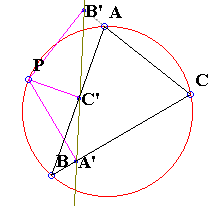# Simson Line: What is it? A Mathematical Droodle

28 November 2015, Created with GeoGebra

Explanation### Theorem (William Wallace, 1797)

The pedal triangle of a point P with respect to ΔABC degenerates into a straight line iff P lies on the circumcircle of ΔABC.

(The line is known as Simson line, or just plain simson. The theorem is often called Simson-Wallace Theorem.)With a reference to the above diagram (other cases of relative point positions are similar), let P lie on the circumcircle of ΔABC. Because of the right angles at A', B' and C' there are several cyclic quadrilaterals. In other words, P lies on the circumcircles of triangles A'BC', A'B'C and AB'C'. Since P is concyclic with (of course) ABC and A'BC',

∠APC = B = ∠A'PC'.

∠A'PC = ∠APC'.

P is also concyclic with A', C and B'. Therefore,

∠A'PC = ∠A'B'C.

Similarly, since P is concyclic with A, B' and C',

∠APC' = ∠AB'C'.

Thus

∠A'B'C = ∠AB'C',

which exactly means that the points A', B' and C' are collinear - the pedal triangle degenerates into a line. And it should be noted that the steps in the above proof are reversible, provided the points are named in an appropriate way.

Note that the converse of the theorem is also true: The other three points of intersection of three concurrent circles are collinear. Then their centers and the point of concurrency are concyclic.

### References

1. H.S.M. Coxeter, S.L. Greitzer, Geometry Revisited, MAA, 1967### Simson Line - the simson

• Simson Line
• Three Concurrent Circles
• 9-point Circle as a locus of concurrency
• Miquel's Point
• Circumcircle of Three Parabola Tangents
• Angle Bisector in Parallelogram
• Simsons and 9-Point Circles in Cyclic Quadrilateral
• Reflections of a Point on the Circumcircle
• Simsons of Diametrically Opposite Points
• Simson Line From Isogonal Perspective
• Pentagon in a Semicircle
• Simson Line in Disguise
• Two Simsons in a Triangle
• Carnot's Theorem
• A Generalization of Simson Line